# Chapter 3 Pair of Linear Equation in Two Variables NCERT Exemplar Solutions Exercise 3.2 Class 10 Maths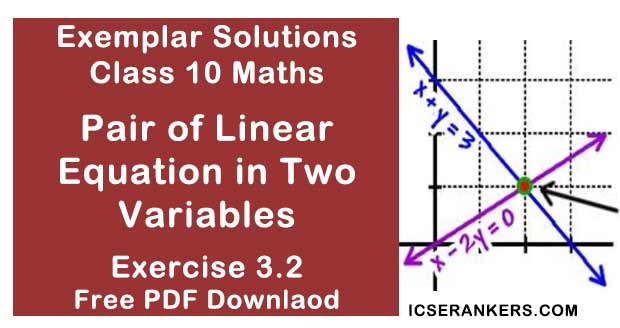Chapter Name NCERT Maths Exemplar Solutions for Chapter 3 Pair of Linear Equation in Two Variables Exercise 3.2 Book Name NCERT Exemplar for Class 10 Maths Other Exercises Exercise 3.1Exercise 3.3Exercise 3.4 Related Study NCERT Solutions for Class 10 Maths

### Exercise 3.2 Solutions

1. Do the following pair of linear equations have no solution? Justify your answer.
(i) 2x + 4y = 3
12y + 6x = 6

(ii) x = 2y
y = 2x

(iii) 3x + y – 3 = 0
2x + 2/3y = 2
Solution
(i) Yes
According to question,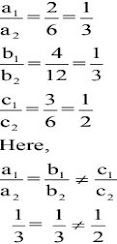Hence, the pair of linear equations has no solutions.

(ii) No,
According to question,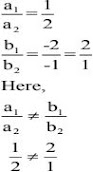Hence the pair of linear equations have unique solutions.

(iii) No
According to question,
a1 = 3,
1 = 1,
c1 = – 3,
a2 = 2,
b2 = 2/3,
c2 = – 2,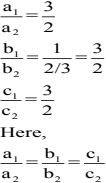So, the pair of linear equations are coincident.

2. Do the following equations represent a pair of coincident lines? Justify your answer.
(i) 3x + 1/7y = 3
7x + 3y = 7

(ii) –2x – 3y = 1
6y + 4x = – 2

(iii) x/2 + y + 2/5 = 0
4x + 8y + 5/16 = 0
Solution
For coincident lines,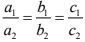(i) No
Given pair of linear equations are:
3x + 1/7y = 3
7x + 3y = 7
We have
a1 = 3,
1 = 1/7,
c1 = – 3,
and
a2 = 7,
b2 = 3,
c2 = – 7,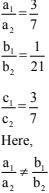The given pair of linear equations has unique solution.

(ii) Yes,
The pair of linear equations are,
– 2x – 3y – 1 = 0 and 4x + 6y + 2 = 0;
We have
a1 = -2,
1 = -3,
c1 = -1,
And
a2 = 4,
b2 = 6,
c2 =  2,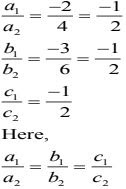The given pair of linear equations is coincident.

(iii) No,
Pair of linear equations are:
x/2 + y + 2/5 = 0
4x + 8y + 5/16 = 0
We have,
a1 =  1/2,
1 =  1,
c1 =  2/5,
And
a2 = 4,
b2 = 8,
c2 =  5/16,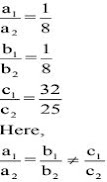Hence, the given pair of linear equations has no solution.

3. Are the following pair of linear equations consistent? Justify your answer.
(i) –3x– 4y = 12
4y + 3x = 12

(ii) (3/5) x – y = 1⁄2
(1/5) x – 3y= 1/6

(iii) 2ax + by = a
ax + 2by – 2a = 0; a, b ≠ 0

(iv) x + 3y = 11
2 (2x + 6y) = 22

Solution
For pair of linear equations to be consistent: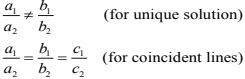(i) No.
The given pair of linear equations
– 3x – 4y – 12 = 0
4y + 3x – 12 = 0
We get,
a1 = -3,
1 = -4,
c1 = -12,
a2 = 3,
b2 = 4,
c2 =  -12,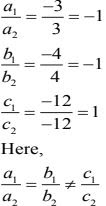The pair of linear equations has no solution, i.e., inconsistent.

(ii) Yes.
The given pair of linear equations
(3/5)x – y = 1⁄2
(1/5)x – 3y = 1/6
We have,
a1 = 3/5,
1 = -1,
c1 = -1/2,
a2 = 1/5,
b2 = 3,
c2 =  -1/6,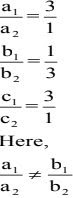The given pair of linear equations has unique solution, i.e., consistent.

(iii) Yes.
The given pair of linear equations –
2ax + by –a = 0
4ax + 2by – 2a = 0
We have,
a1 = 2a,
1 = b,
c1 = -a,
a2 = 4a,
b2 = 2b,
c2 =  -2a,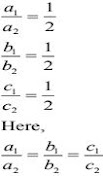The given pair of linear equations has infinitely many solution, i.e., consistent

(iv) No.
The given pair of linear equations
x + 3y = 11
2x + 6y = 11
We have,
a1 = 1,
1 = 3,
c1 = 11,
a2 = 2,
b2 = 6,
c2 =  11,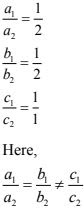The given pair of linear equations has no solution.

4. For the pair of equations λx + 3y = –7 , 2x + 6y = 14to have infinitely many solutions, the value of λ should be 1. Is the statement true? Give reasons.
Solution
λx + 3y + 7 = 0 .
2x + 6y – 14 = 0
For infinitely many solutions,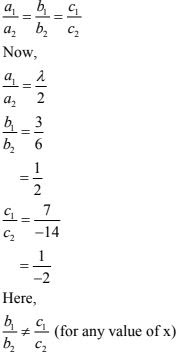So, the given statement is not true.

5. For all real values of c, the pair of equations x – 2y = 8 5x – 10y = c have a unique solution. Justify whether it is true or false.
Solution
(Not true)
System of linear equations are
x – 2y = 8 ...(i)
5x – 10 = c ...(ii)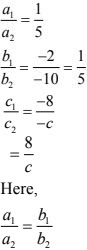Hence, system of linear equations can never have unique solution.
So, the given statement is not true.

6. The line represented by x = 7 is parallel to the x–axis. Justify whether the statement is true or not.
Solution
The line x = 7 is the form of x = a. The graph of the equation is a line parallel to the y–axis.
Hence, the given statement is false.